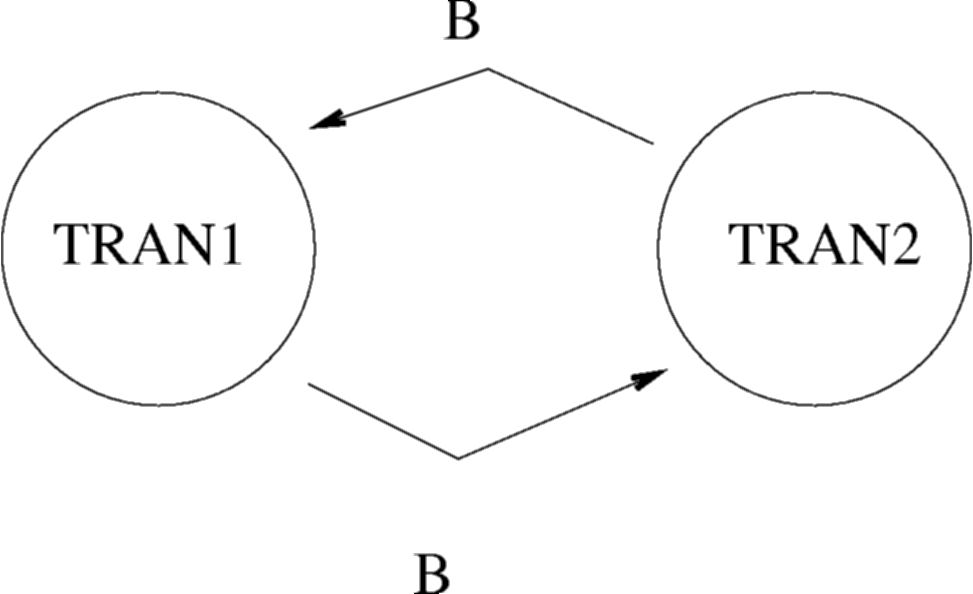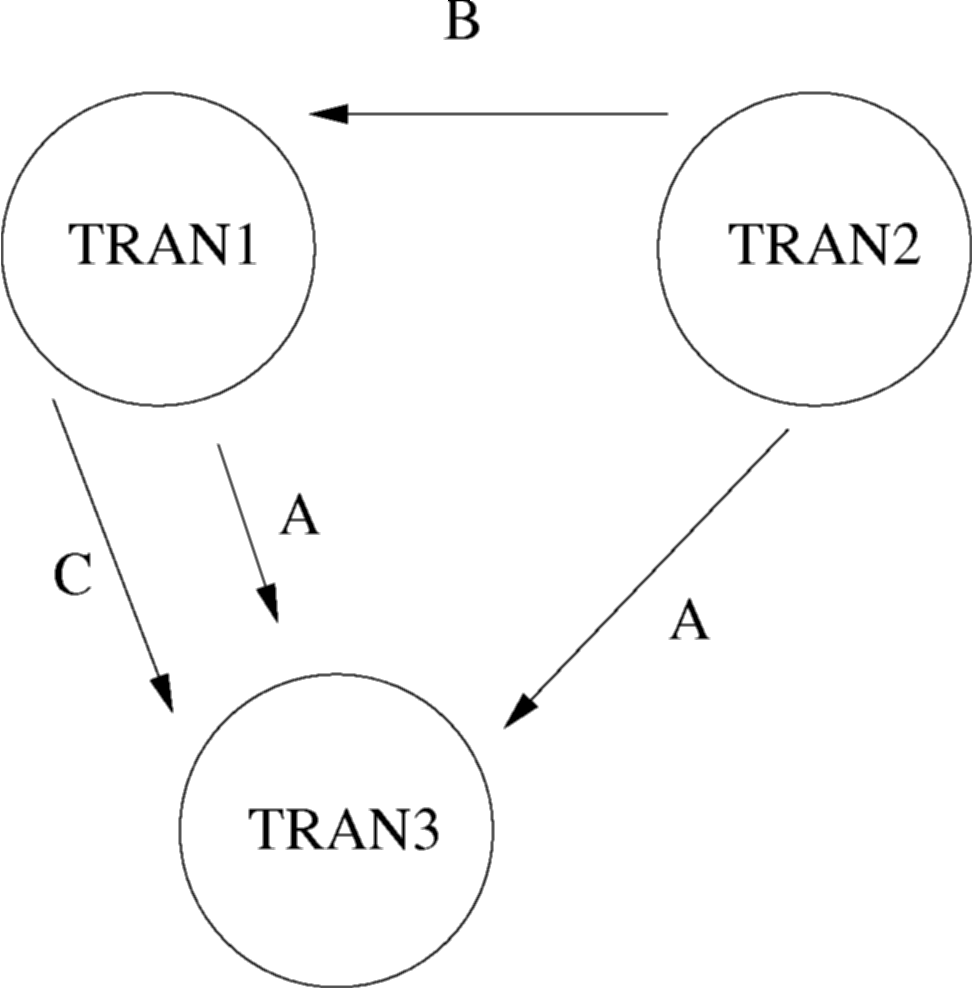Next Page Up One Level

Lecture Slides available: PDF PowerPoint

# Concurrency using Transactions

## Contents

The goal in a `concurrent' DBMS is to allow multiple users to access the database simultaneously without interfering with each other.

A problem with multiple users using the DBMS is that it may be possible for two users to try and change data in the database simultaneously. If this type of action is not carefully controlled, inconsistencies are possible.

To control data access, we first need a concept to allow us to encapsulate database accesses. Such encapsulation is called a `Transaction'.

## Transactions

• Transaction (ACID)
• unit of logical work and recovery
• A - atomicity (for integrity)
• C - consistency preservation
• I - isolation
• D - durability
• Available in SQL
• Some applications require nested or long transactions

After work is performed in a transaction, two outcomes are possible:

• Commit - Any changes made during the transaction by this transaction are committed to the database.
• Abort - All the changes made during the transaction by this transaction are not made to the database. The result of this is as if the transaction was never started.

## Transaction Schedules

A transaction schedule is a tabular representation of how a set of transactions were executed over time. This is useful when examining problem scenarios. Within the diagrams various nomenclatures are used:

• READ(a) - This is a read action on an attribute or data item called `a'.
• WRITE(x,a) - This is a write action on an attribute or data item called `a', where the value `x' is written into `a'.
• tn (e.g. t1,t2,t10) - This indicates the time at which something occurred. The units are not important, but tn always occurs before tn+1.

Consider transaction A, which loads in a bank account balance X (initially 20) and adds 10 pounds to it. Such a schedule would look like this:

 Time Transaction A t1 TOTAL:=READ(X) t2 TOTAL:=TOTAL+10 t3 WRITE(TOTAL,X)

Now consider that, at the same time as transaction A runs, transaction B runs. Transaction B gives all accounts a 10% increase. Will X be 32 or 33?

TimeTransaction AValue
TOTAL
Transaction BValue
BALANCE
t3TOTAL:=TOTAL+1030
t4WRITE(TOTAL,X)30
t5  BALANCE:=BALANCE*110%22
t6  WRITE(BALANCE,X)22

Whoops... X is 22! Depending on the interleaving, X can also be 32, 33, or 30. Lets classify erroneous scenarios.

## Lost Update scenario.

 Time Transaction A Transaction B t1 X=READ(R) t2 Y=READ(R) t3 WRITE(X,R) t4 WRITE(Y,R)

Transaction A's update is lost at t4, because Transaction B overwrites it. B missed A's update at t3 as it got the value of R at t2.

## Uncommitted Dependency

 Time Transaction A Transaction B t1 WRITE(X,R) t2 Y=READ(R) t3 ABORT

Transaction A is allowed to READ (or WRITE) item R which has been updated by another transaction but not committed (and in this case ABORTed).

## Inconsistency

 Time X Y Z Transaction A Transaction B Action SUM t1 40 50 30 SUM:=READ(X) 40 t2 40 50 30 SUM+=READ(Y) 90 t3 40 50 30 ACC1=READ(Z) t4 40 50 20 WRITE(ACC1-10,Z) t5 40 50 20 ACC2=READ(X) t6 50 50 20 WRITE(AC2+10,X) t7 50 50 20 COMMIT t7 50 50 20 SUM+=READ(Z) 110 SUM should have been 120...

## Serialisability

• A `schedule' is the actual execution sequence of two or more concurrent transactions.
• A schedule of two transactions T1 and T2 is `serialisable' if and only if executing this schedule has the same effect as either T1;T2 or T2;T1.

## Precedence Graph

In order to know that a particular transaction schedule can be serialized, we can draw a precedence graph. This is a graph of nodes and vertices, where the nodes are the transaction names and the vertices are attribute collisions.

The schedule is said to be serialised if and only if there are no cycles in the resulting diagram.

## Precedence Graph : Method

To draw one;

• Draw a node for each transaction in the schedule
• Where transaction A writes to an attribute which transaction B has read from, draw an line pointing from B to A.
• Where transaction A writes to an attribute which transaction B has written to, draw a line pointing from B to A.
• Where transaction A reads from an attribute which transaction B has written to, draw a line pointing from B to A.

## Example 1

Consider the following schedule:

TimeTRAN1TRAN2
t5WRITE(x,B)
t6  WRITE(y,B)## Example 2

Consider the following schedule:

TimeTRAN1TRAN2TRAN3